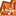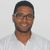# App Development

Discussion board where members can learn more about Qlik Sense App Development and Usage.

Announcements
Welcome to Qlik Community! Check out our new navigation! FIND OUT MORE
cancel
Showing results for
Did you mean:Creator

## Quarter Calculations

Hi all,

As many other people, I am also new in the app development with Qlik Sense and I am currently experiencing an issue, concerning quarters and some calculations based on them.

My data is such that it is not possible to create a calendar in Qlik Sense and I need to use the Quarter field that I already have in my excel table.

The Quarter field is in the format - '2013Q1' , '2013Q2', '2013Q3','2013Q4', '2014Q1', etc...

I have altered the script a bit in order to create another field (NumericQuarterField), based on the initial Quarter field that I have and I have removed the "Q" so that it can be a numeric field, so on the currently field I have this format - '20131' , '20132', '20133','20134', '20141', etc...

I have used that numeric field to do a couple of calculations, for example Year over Year growth:

(sum({<NumericQuarterField={\$(=max(NumericQuarterField))}>} Data) - sum({<NumericQuarterField={\$(=max(NumericQuarterField)-10)}>} Data))/ sum({<NumericQuarterField={\$(=max(NumericQuarterField)-10)}>} Data)

The calculation works pretty fine and shows correct results, but then the issue comes when I add a filter, based on the Quarter field (the one that has 'Q' in the name of the Quarter) because if you make a selection in the filter pane, for instance - "2013Q2", Qlik Sense doesn't actually recognize that this is "20132" and shows null values.

As I am pretty sure that the issue comes from the 'max' function I have added in order for the calculation to be performed, do you have any other suggestions on how I can alter the calculation expression so that it can work with the filter pane based on the Quarter field?

Labels (3)

• ### quarter

1 Solution

Accepted SolutionsPartner - Contributor III

Try ...
(sum({<NumericQuarterField={\$(=max(NumericQuarterField))},Quarter=>} Data)

-

sum({<NumericQuarterField={\$(=max(NumericQuarterField)-10)},Quarter=>} Data))

/ sum({<NumericQuarterField={\$(=max(NumericQuarterField)-10)},Quarter=>} Data)

Regards,

GAbriel

3 RepliesMVP

can you share a sample data?

Regards,
Taoufiq ZARRA

"Please LIKE posts and "Accept as Solution" if the provided solution is helpful "

(you can mark up to 3 "solutions") 😉Partner - Contributor III

Try ...
(sum({<NumericQuarterField={\$(=max(NumericQuarterField))},Quarter=>} Data)

-

sum({<NumericQuarterField={\$(=max(NumericQuarterField)-10)},Quarter=>} Data))

/ sum({<NumericQuarterField={\$(=max(NumericQuarterField)-10)},Quarter=>} Data)

Regards,

GAbrielCreator
Author

Hello Gabriel,

Perfect! Thank you very much, it worked flawlessly!

Have a wonderful day!Tags
Community Browser